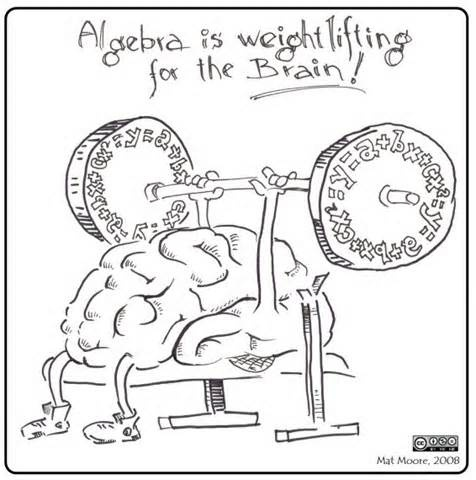Math/AlgebraOverview

The 8th grade math curriculum consists of the following topics

 Real Numbers Scientific Notation Functions Volume Irrational Numbers Proportional Relationships Linear Relationships Scatterplots Exponential Expressions Linear Equations Object Transformations Two-Way Tables Cube and Square Roots Systems of Equations Pythagorean Theorem Linear/Non-Linear Models

In addition, students taking the Algebra I will study:

 Radicals Inequalities Writing Linear Equations Polynomials Systems of Inequalities Statistics and Probability Factoring Domain and Range Rational Expressions

Supplies

All students are expected to bring the following every day:

Charged Chromebook

Textbook

1.5"-2" binder

Pencils

Looking forward to memorable year.  Please e-mail me if you have any questions!  Enjoy the rest of your summer.